Homework Help Question & Answers

# A rocket is launched from rest with a time-dependent acceleration a(t) = (p – rt), where...

A rocket is launched from rest with a time-dependent acceleration a(t) = (p – rt), where p = 32.00 m/s2 and r = 5.00 m/s3. Find the maximum velocity of the rocket

#### Homework Answers

Answer #1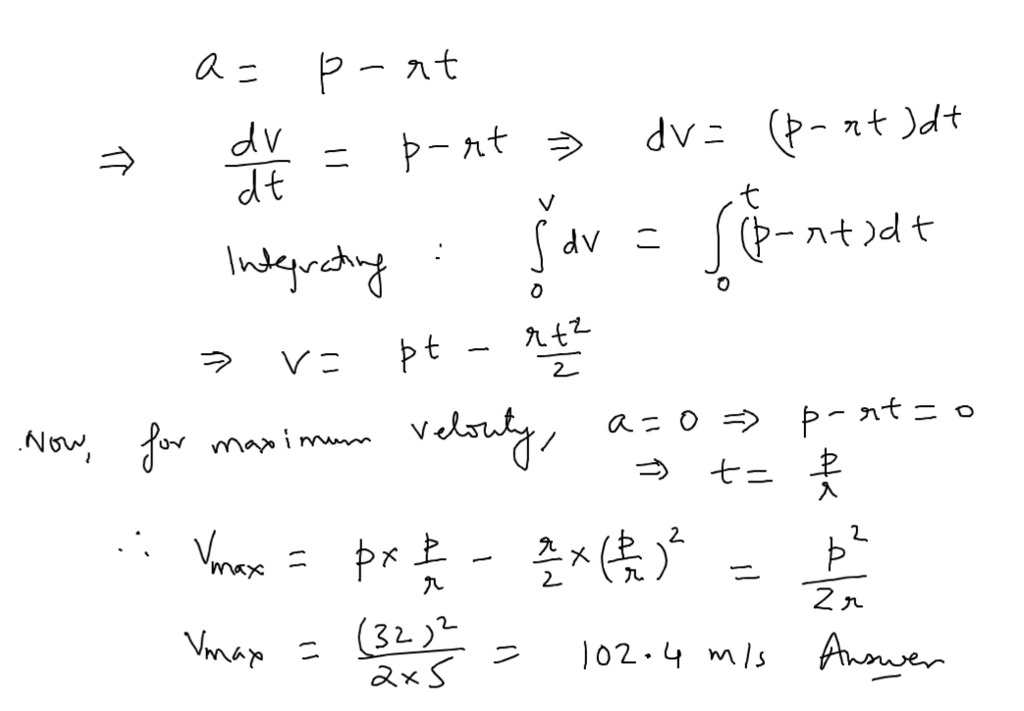Know the answer?
Your Answer:

#### Post as a guest

Your Name:

What's your source?

#### Earn Coin

Coins can be redeemed for fabulous gifts.

Not the answer you're looking for? Ask your own homework help question. Our experts will answer your question WITHIN MINUTES for Free.
Similar Homework Help Questions
• ### A rocket is launched from rest with a time-dependent acceleration a(t) = (p – rt), where...

A rocket is launched from rest with a time-dependent acceleration a(t) = (p – rt), where p = 15.00 m/s2 and r = 3.00 m/s3. Find the maximum velocity of the rocket.

• ### A rocket is launched from rest with a time-dependent upward acceleration a(t) = k – ft....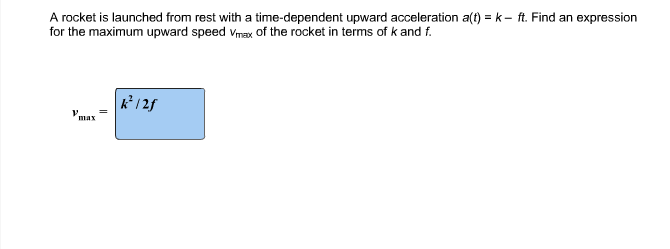A rocket is launched from rest with a time-dependent upward acceleration a(t) = k – ft. Find an expression for the maximum upward speed vmax of the rocket in terms of k and f. A rocket is launched from rest with a time-dependent upward acceleration at) k- ft. Find an expression for the maximum upward speed Vmax of the rocket in terms of k and f /2f max

• ### A rocket blasts off vertically from rest on the launch pad with a constant upward acceleration...

A rocket blasts off vertically from rest on the launch pad with a constant upward acceleration of 2.60 m/s2 .At 30.0 s after blastoff, the engines suddenly fail, and the rocket begins free fall. What is the height of the rocket when the engine fails? Find the magnitudes of the rocket's velocity and acceleration at its highest point. How long after it was launched will the rocket fall back to the launch pad?

• ### A rocket, initially at rest on the ground, accelerates straight upward from rest with constant acceleration...

A rocket, initially at rest on the ground, accelerates straight upward from rest with constant acceleration 53.9 m/s2m/s2 . The acceleration period lasts for time 8.00 ss until the fuel is exhausted. After that, the rocket is in free fall. Find the maximum height ymax reached by the rocket. Ignore air resistance and assume a constant acceleration due to gravity equal to 9.80 m/s2 . Write your answer numerically in units of meters.

• ### A rocket, initially at rest on the ground, accelerates straight upward from rest with constant acceleration...

A rocket, initially at rest on the ground, accelerates straight upward from rest with constant acceleration 29.4m/s2 . The acceleration period lasts for time 9.00s until the fuel is exhausted. After that, the rocket is in free fall. Find the maximum height ymax reached by the rocket. Ignore air resistance and assume a constant acceleration due to gravity equal to 9.80 m/s2 . Write your answer numerically in units of meters.

• ### A. If a rocket was launched vertically from rest with an acceleration of 15 m/s² for...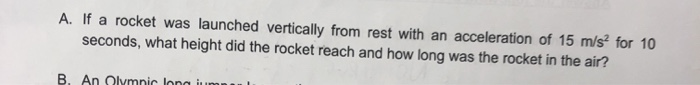A. If a rocket was launched vertically from rest with an acceleration of 15 m/s² for 10 seconds, what height did the rocket reach and how long was the rocket in the air?

• ### "A rocket is launched vertically from rest with a constant upward acceleration of 2.59 m/s2 ....

"A rocket is launched vertically from rest with a constant upward acceleration of 2.59 m/s2 . Suddenly 19.9 s after launch, an unneeded fuel tank is jettisoned by shooting it away from the rocket. A crew member riding in the rocket measures that the initial speed of the tank is 20.4 m/s and that it moves perpendicular to the rocket's path. The fuel tank feels no appreciable air resistance and feels only the force of gravity once it leaves the...

• ### A rocket, initially at rest on the ground, accelerates straight upward from rest with constant acceleration...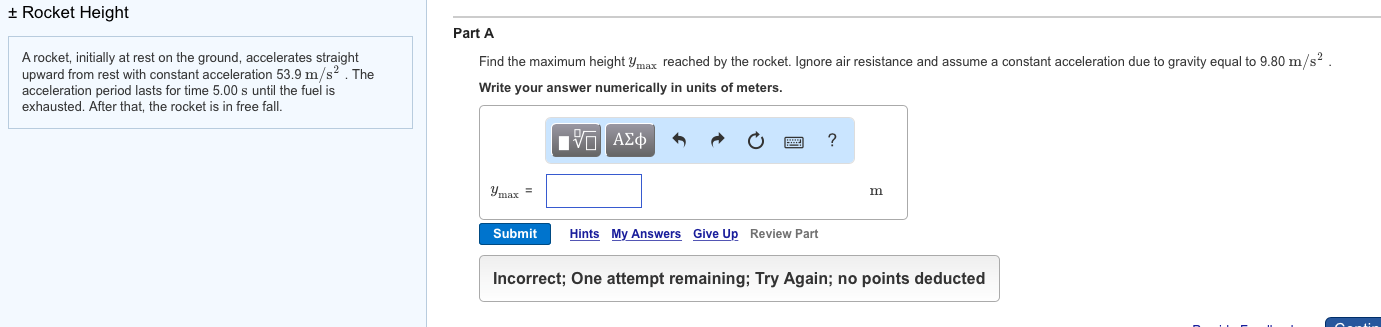A rocket, initially at rest on the ground, accelerates straight upward from rest with constant acceleration 53.9 m/s^2. The acceleration period lasts for time 5.00 s until the fuel is exhausted. After that, the rocket is in free fall. Find the maximum height y_max reached by the rocket. Ignore air resistance and assume a constant acceleration due to gravity equal to 9.80 m/s^2. Write your answer numerically in units of meters.

• ### 1. A rocket is launched straight up with constant unknown acceleration a). A time t) after...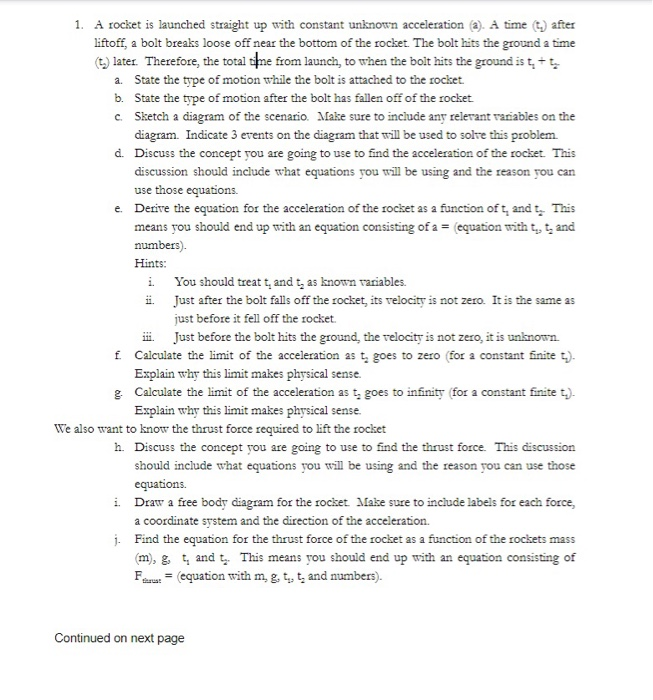1. A rocket is launched straight up with constant unknown acceleration a). A time t) after liftoff, a bolt breaks loose off near the bottom of the rocket. The bolt hits the ground a time (t) later. Therefore, the total ne from launch, to when the bolt hits the ground ist, + t a. State the type of motion while the bolt is attached to the rocket. b. State the type of motion after the bolt has fallen off of...

• ### Problem 4.7 Constants| Periodic Table Part A A model rocket is launched from rest with an...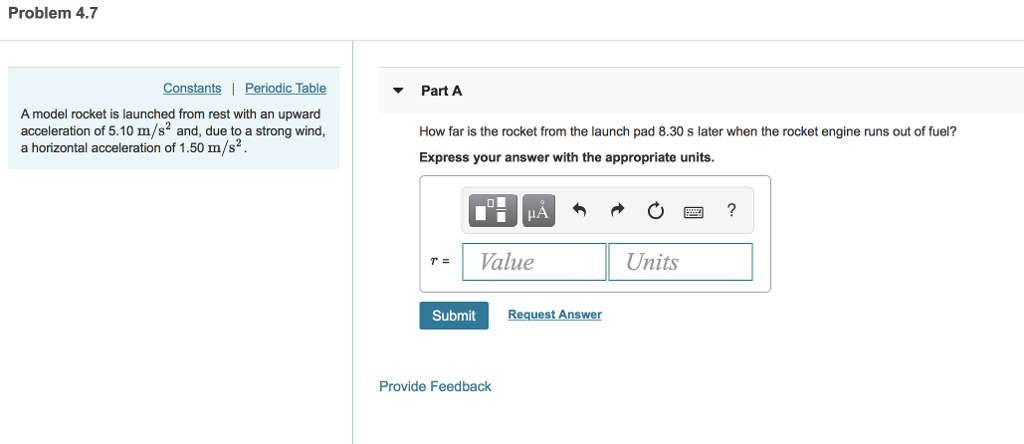Problem 4.7 Constants| Periodic Table Part A A model rocket is launched from rest with an upward acceleration of 5.10 m/s2 and, due to a strong wind a horizontal acceleration of 1.50 m/s2 How far is the rocket from the launch pad 8.30 s later when the rocket engine runs out of fuel? Express your answer with the appropriate units. rValue Units Submit Request Answer Provide Feedback

Free Homework App

Scan Your Homework
to Get Instant Free Answers
Need Online Homework Help?

Get Answers For Free
Most questions answered within 3 hours.